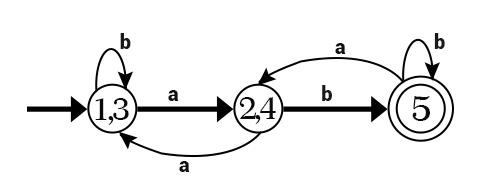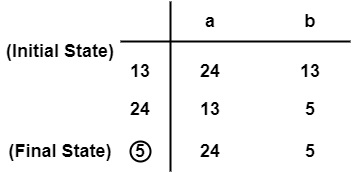# What is Deterministic Finite Automata (DFA)?

Deterministic means that on each input there is one and only one state to which the automata can have the transition from its current state. In deterministic finite automata, the head can move only in one direction to scan the input tape symbols. But in the case of two-way, finite automata on scanning an input symbol the head of the tape may move in right or left from its current position.

A deterministic finite automata is a set of 5 tuples and defined as

M=(Q,Σ,$\delta$,q0,F) where,

• Q: A non-empty finite set of states present in the finite control (q0,q1,q2).

• $\sum$:A Non-empty finite set of input symbols.

• $\delta$: It is a transition function that takes two arguments, a state, and an input symbol, it returns a single state represented by ∴ $\delta$:Q x $\sum$ →Q. Let q is the state and a be the input symbol passed to the transition function. $\delta$(q,a)=q. q is the output of the function, which may be the same or new state.

• q0 ∈ Q is the initial state.

• F $\subseteq$ Q is the set of final states.

Example − Minimize the following DFA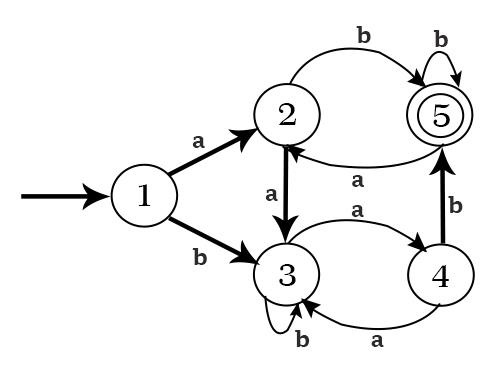Solution:

• Make a transition Table.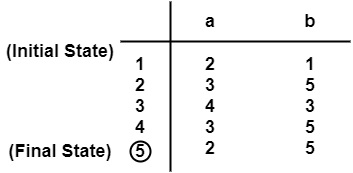• π0= {{5}}, {1, 2, 3, 4}}
• For input a, on {1, 2, 3, 4} of π0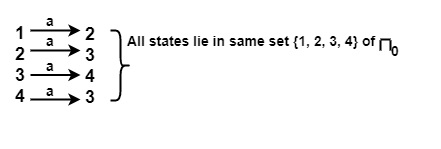• For input b, on {1, 2, 3, 4} of π0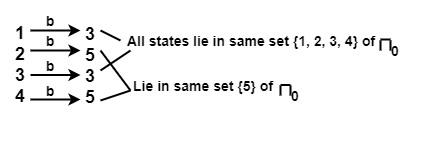∴ {1, 2, 3, 4} will be split into {1, 3} and {2, 4}

∴ π1={{5},{1,3},{2,4}}

• For input symbol a on {1, 3} of π1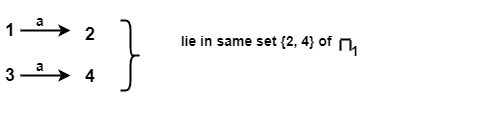Similarly for input symbol a on {2, 4} of π1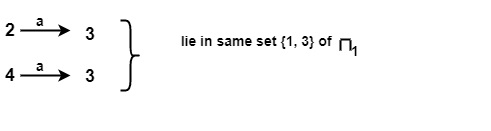• For input symbol b on {1, 3} of π1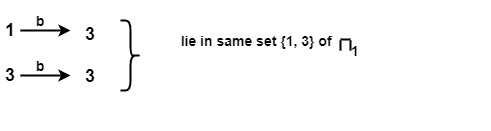Similarly for input symbol b on {2, 4} of π1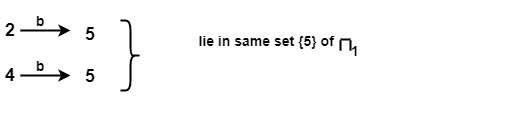Subset in π1 i.e., {1, 3} & {2, 4} will not be splitted.

πfinal= {{5}, {1, 3}, {2, 4}}

There will be 3 states of DFA.

{5}, {1, 3} and {2, 4}

Minimized DFA will be −